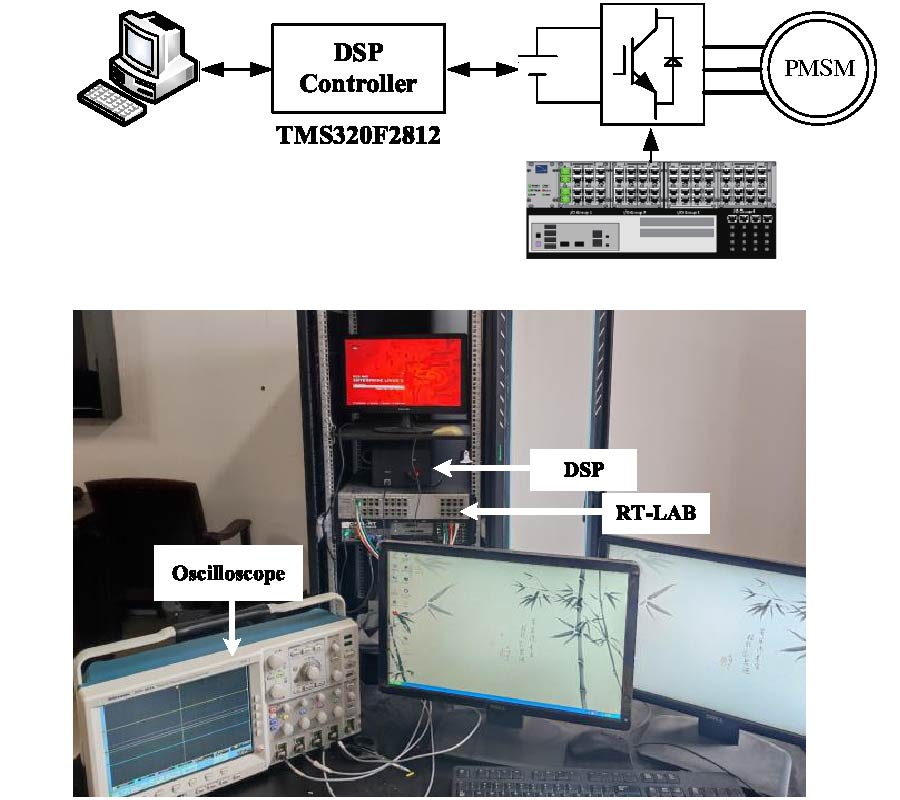Vol. 131
Latest Volume
All Volumes
All Issues
2023-03-27
Dual Cost Function Model Predictive Control for PMSM
By
Progress In Electromagnetics Research C, Vol. 131, 171-184, 2023
Abstract
In model predictive current control (MPCC), in order to reduce the switching frequency, the number of switching changes is introduced into the cost function. But it will lead to the complexity of weight coefficient adjustment. To solve the problem, a dual cost function model predictive control (DCF-MPC) strategy for permanent magnet synchronous motor (PMSM) is proposed. First, the dual cost function is established, and the cost function g1 first screens out the combination of two or three voltage vectors which minimizes the current steady-state error. Then, the cost function g2 selects the voltage vector combination that minimizes the number of switching changes from the selected voltage vector combinations in g1 as the optimal voltage vector combination. Finally, the experiment shows that compared with the traditional single cost function, the proposed method eliminates the weight coefficient of MPCC, simplifies the system structure and reduces the amount of calculation. Moreover, it suppresses the stator current ripple, reduces the harmonic content of three-phase current, and has better steady-state and dynamic performance under different working conditions.
Citation
Dingdou Wen Wenting Zhang Zhe Li Zhongjian Tang Yang Zhang Yun Ling , "Dual Cost Function Model Predictive Control for PMSM," Progress In Electromagnetics Research C, Vol. 131, 171-184, 2023.
doi:10.2528/PIERC23011502
http://www.jpier.org/PIERC/pier.php?paper=23011502
References

Download Full Article (24) View Full Article